## Example Questions

### Example Question #1 : Algebraic Fractions

Which of the following are answers to the equation below?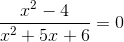1.2.3.2 only

2 and 3

3 only

1 only

1, 2, and 3

3 only

Explanation:

Given a fractional algebraic equation with variables in the numerator and denominator of one side and the other side equal to zero, we rely on a simple concept.  Zero divided by anything equals zero. That means we can focus in on what values make the numerator (the top part of the fraction) zero, or in other words,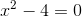The expression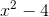is a difference of squares that can be factored as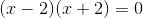Solving this forgives eitheror.  That means either of these values will make our numerator equal zero.  We might be tempted to conclude that both are valid answers.  However, our statement earlier that zero divided by anything is zero has one caveat. We can never divide by zero itself.  That means that any values that make our denominator zero must be rejected.  Therefore we must also look at the denominator.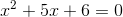The left side factors as follows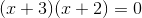This means that ifisor, we end up dividing by zero.  That means thatcannot be a valid solution, leavingas the only valid answer.  Therefore only #3 is correct.

### Example Question #41 : Algebraic Fractions

Which of the following provides the complete solution set for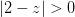?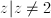No solutions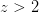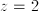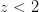Explanation:

The absolute value will always be positive or 0, therefore all values of z will create a true statement as long as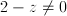. Thus all values except for 2 will work.

### Example Question #1 : How To Find Excluded Values

If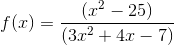then which values ofcannot exist?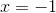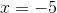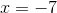Explanation:

The denominator of a fraction can never be equal to 0.

Therefore, to find out what x cannot be equal to, we must factor the denominator, and determine what values of x would make it equal to 0.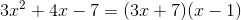Therefore,and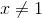.

### Example Question #1 : Algebraic Fractions

If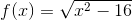then which cannot be anvalue?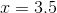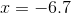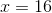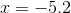Explanation:

You cannot take the square root of a negative number.

Setting up the inequality we get: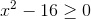Solving forwe get: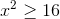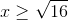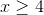Therefore any value less than four will not work,.

Another approach is to plug in each of the possible values.

When plugged intoall of the answers give us a value greater than or equal to 0, except for, which gives us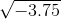.

### Example Question #1 : How To Find Excluded Values

Find the excluded values of the following algebraic fraction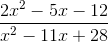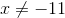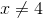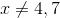The numerator cancels all the binomials in the denomniator so ther are no excluded values.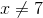Explanation:

To find the excluded values of a algebraic fraction you need to find when the denominator is zero. To find when the denominator is zero you need to factor it. This denominator factors into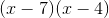so this is zero when x=4,7 so our answer is### All GRE Math Resources